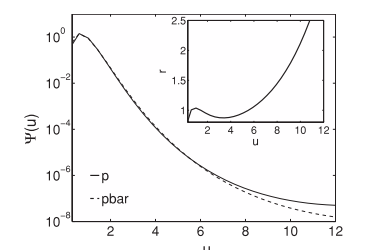# How to insert one small graph into a big graph in ROOT?

See the attachment.
This graph is done by matlab. How can I insert a small graph into a big graph in ROOT ? Could sb. give me some hints? I have looked at the tutorial, but there is only the case that the two graphs are in different y scale.simply create a new pad inside the main pad as shown in the example below

Rene

```void twopads() { TCanvas *c1 = new TCanvas("c1"); TH1F *h1 = new TH1F("h1","test1",100,-4,4); h1->FillRandom("gaus"); h1->Draw(); TPad *subpad = new TPad("subpad","",0.6,0.3,0.95,0.65); subpad->Draw(); subpad->cd(); TH1F *h2 = new TH1F("h2","test2",100,-2,4); h2->FillRandom("gaus"); h2->Draw(); } ```

Thank you very much, Rene.